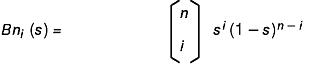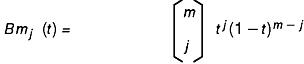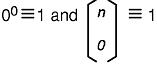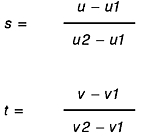[ Previous | Next | Contents | Glossary | Home | Search ]
OpenGL 1.1 for AIX: Reference Manual

## glMap2 Subroutine

### Purpose

Defines a 2-dimensional (2D) evaluator.

### Library

OpenGL C bindings library: libGL.a

### C Syntax

```void glMap2d(GLenum Target,
GLdouble u1,
GLdouble u2,
GLint uStride,
GLint uOrder,
GLdouble v1,
GLdouble v2,
GLint vStride,
GLint vOrder,
const GLdouble *Points)```
```void glMap2f(GLenum Target,
GLfloat u1,
GLfloat u2,
GLint uStride,
GLint uOrder,
GLfloat v1,
GLfloat v2,
GLint vStride,
GLint vOrder,
const GLfloat *Points)```

### Parameters

 Target Specifies the kind of values that are generated by the evaluator. The following symbolic constants are accepted: GL_MAP2_VERTEX_3 GL_MAP2_VERTEX_4 GL_MAP2_INDEX GL_MAP2_COLOR_4 GL_MAP2_NORMAL GL_MAP2_TEXTURE_COORD_1 GL_MAP2_TEXTURE_COORD_2 GL_MAP2_TEXTURE_COORD_3 GL_MAP2_TEXTURE_COORD_4 u1, u2 Specify a linear mapping of u, as presented to glEvalCoord2, to u1, one of the two variables that is evaluated by the equations specified by this subroutine. uStride Specifies the number of floats or doubles between the beginning of control point Rij and the beginning of control point R(i+1)j, where i and j are the u and y control-point indexes, respectively. This allows control points to be embedded in arbitrary data structures. The only constraint is that the values for a particular control point must occupy contiguous memory locations. uOrder Specifies the dimension of the control point array in the u axis. Must be positive. v1, v2 Specify a linear mapping of v, as presented to glEvalCoord2, to v1, one of the two variables that is evaluated by the equations specified by this subroutine. vStride Specifies the number of floats or doubles between the beginning of control point Rij and the beginning of control point Ri(j+1), where i and j are the u and v control point indexes, respectively. This allows control points to be embedded in arbitrary data structures. The only constraint is that the values for a particular control point must occupy contiguous memory locations. vOrder Specifies the dimension of the control point array in the v axis. Must be positive. Points Specifies a pointer to the array of control points.

### Description

Evaluators provide a way to use polynomial or rational polynomial mapping to produce vertices, normals, texture coordinates, and colors. The values produced by an evaluator are sent on to further stages of GL processing just as if they had been presented using the glVertex, glNormal, glTexCoord, and glColor subroutines, except that the generated values do not update the current normal, texture coordinates, or color.

All polynomial or rational polynomial splines of any degree (up to the maximum degree supported by the GL implementation) can be described using evaluators. These include almost all surfaces used in computer graphics, such as B-spline surfaces, non-uniform rational B-spline surfaces (NURBS), and Bezier surfaces.

Evaluators define surfaces based on bivariate Bernstein polynomials. Define p(s, t) as follows:

```Let p(s, t) = Bn0Bm0R00 + Bn1Bm0R01 + . . . + BnnBm0Rn0
+Bn0Bm1R01 + . . . + BnnBm1Rn1
.
.
.
+Bn0BmmR0m + . . . + BnnBmmRnm```

where Rij is a control point, Bni (s) is the ith Bernstein polynomial of degree:

`n (uOrder = n +1)`

See the following figure:and Bmj (t) is the jth Bernstein polynomial of degree:

`m (vOrder = m + 1)`

See the following figure:See the following figure:The glMap2 subroutine is used to define the basis and to specify what kind of values are produced. Once defined, a map can be enabled and disabled by calling the glEnable and glDisable subroutines with the map name, which is one of the nine predefined values for the Target parameter. When the glEvalCoord2 subroutine presents values u and v, the bivariate Bernstein polynomials are evaluated using s and t, as in the following figure:The Target parameter specifies a symbolic constant that indicates what kind of control points are provided in the Points parameter, and what output is generated when the map is evaluated. It can assume one of the following nine predefined values:

 GL_MAP2_VERTEX_3 Each control point is three floating-point values representing x, y, and z. Internal glVertex3 subroutines are generated when the map is evaluated. GL_MAP2_VERTEX_4 Each control point is four floating-point values representing x, y, z, and w. Internal glVertex4 subroutines are generated when the map is evaluated. GL_MAP2_INDEX Each control point is a single floating-point value representing a color index. Internal glIndex subroutines are generated when the map is evaluated. However, the current index is not updated with the value of these glIndex subroutines. GL_MAP2_COLOR_4 Each control point is four floating-point values representing red, green, blue, and alpha. Internal glColor4 subroutines are generated when the map is evaluated. However, the current color is not updated with the value of these glColor4 subroutines. GL_MAP2_NORMAL Each control point is three floating-point values representing the x, y, and z components of a normal vector. Internal glNormal subroutines are generated when the map is evaluated. However, the current normal is not updated with the value of these glNormal subroutines. GL_MAP2_TEXTURE_COORD_1 Each control point is a single floating-point value representing the s texture coordinate. Internal glTexCoord1 subroutines are generated when the map is evaluated. However, the current texture coordinates are not updated with the value of these glTexCoord subroutines. GL_MAP2_TEXTURE_COORD_2 Each control point is two floating-point values representing the s and t texture coordinates. Internal glTexCoord2 subroutines are generated when the map is evaluated. However, the current texture coordinates are not updated with the value of these glTexCoord subroutines. GL_MAP2_TEXTURE_COORD_3 Each control point is three floating-point values representing the s, t, and r texture coordinates. Internal glTexCoord3 subroutines are generated when the map is evaluated. However, the current texture coordinates are not updated with the value of these glTexCoord subroutines. GL_MAP2_TEXTURE_COORD_4 Each control point is four floating-point values representing the s, t, r, and q texture coordinates. Internal glTexCoord4 subroutines are generated when the map is evaluated. However, the current texture coordinates are not updated with the value of these glTexCoord subroutines.

The uStride, uOrder, vStride, vOrder, and Points parameters define the array addressing for accessing the control points. The Points parameter is the location of the first control point, which occupies one, two, three, or four contiguous memory locations, depending on which map is being defined. There are uOrder times vOrder control points in the array. The uStride parameter tells how many float or double locations are skipped to advance the internal memory pointer from control point Rij to control point R(i+1)j. The vStride parameter tells how many float or double locations are skipped to advance the internal memory pointer from control point Rij to control point Ri(j+1).

### Notes

For all GL subroutines that accept pointers to data, it is as if the contents of Points were copied by glMap2 before it returned. Changes to the contents of Points have no effect after glMap2 is called.

### Errors

 GL_INVALID_ENUM Target is not an accepted value. GL_INVALID_VALUE u1 is equal to u2, or if v1 is equal to v2. GL_INVALID_VALUE uStride or vStride is less than the number of values in a control point. GL_INVALID_VALUE uOrder or vOrder is less than one or greater than GL_MAX_EVAL_ORDER. GL_INVALID_OPERATION The glMap2 subroutine is called between a call to glBegin and the corresponding call to glEnd.

### Associated Gets

Associated gets for the glMap2 subroutine are as follows. (See the glGet subroutine for more information.)

glGetMap

glGet with argument GL_MAX_EVAL_ORDER

glIsEnabled with argument GL_MAP2_VERTEX_3

glIsEnabled with argument GL_MAP2_VERTEX_4

glIsEnabled with argument GL_MAP2_INDEX

glIsEnabled with argument GL_MAP2_COLOR_4

glIsEnabled with argument GL_MAP2_NORMAL

glIsEnabled with argument GL_MAP2_TEXTURE_COORD_1

glIsEnabled with argument GL_MAP2_TEXTURE_COORD_2

glIsEnabled with argument GL_MAP2_TEXTURE_COORD_3

glIsEnabled with argument GL_MAP2_TEXTURE_COORD_4.

### Files

 /usr/include/GL/gl.h Contains C language constants, variable type definitions, and ANSI function prototypes for OpenGL.

### Related Information

The glBegin or glEnd subroutine, glColor subroutine, glEnable or Disable subroutine, glEvalCoord subroutine, glEvalMesh subroutine, glEvalPoint subroutine, glGetMap subroutine, glIndex subroutine, glMap1 subroutine, glMapGrid subroutine, glNormal subroutine, glTexCoord subroutine, glVertex subroutine.

[ Previous | Next | Contents | Glossary | Home | Search ]